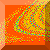# 1. Introduction

The study of chaotic behavior in scattering problems has, in recent years, uncovered many fundamental properties and has greatly enriched our understanding of the dynamics of these systems [Noid et al., 1986; Jung, 1986; Eckhardt, 1988; Hénon, 1988; Bleher et al., 1990; Kovács, 1990; Ding et al., 1990; Smilansky, 1992; Ott & Tél, 1993; Ott, 1993; Lai et al., 1993]. In scattering problems, one is typically interested in the behavior of the scattering function which embodies the relationship between the input variable(s) characterizing the initial condition of the incident trajectory and the output variable(s) characterizing the trajectory after it exits the scattering region. In many cases, the scattering function may have exceedingly complex behavior, where the function is singular on a fractal set of values of the input variables. This type of behavior is indicative of the presence of a chaotic set in the dynamics [Noid et al., 1986; Jung, 1986; Hénon, 1988; Bleher et al., 1990; Kovács, 1990; Ding et al., 1990; Troll, 1991], and near the singular set tiny changes in the input variables can produce drastic changes in the output variables. Scattering systems which exhibit this property are now commonly referred to as ``chaotic scattering'' systems.

In a study by Bleher et al. , it was found that if a trajectory of a chaotic scattering system can leave the scattering region in one of several different ways, then the space of initial conditions corresponding to the various exit modes may be separated by a boundary which is fractal. Unlike dissipative dynamical systems, where the attractors are generally bounded, the ``attractors'' in these Hamiltonian systems are located at infinity. Instead of representing where the trajectories eventually end up, these ``attractors'' represent how the trajectories exit the system; specifically, they correspond to which exit the trajectories took to leave the system. (We assume that almost all trajectories will eventually escape from the system, and that there exists a set of trajectories which stay in the scattering region for all time, but its Lebesgue measure is zero.) We call the collection of initial conditions which eventually leave the system through a particular exit the exit basin of that exit. The existence of fractal boundaries separating the various basins has an important effect on the system: final state sensitivity [Grebogi et al., 1983b; MacDonald et al., 1985]. That is, in the presence of small uncertainty in the input, the measure of input variable values for which it is not possible to predict the ultimate exiting mode is anomalously large (as compared to the case with nonfractal boundaries).

In this paper, we show that not only are the boundaries separating exit basins typically fractal, but they may also possess the stronger property that any point which is on the boundary of one exit basin is also simultaneously on the boundary of all the other exit basins. This interesting property is known as the Wada property. Wada boundaries are to be contrasted with more usual boundaries such as those on a map showing the countries of Europe: there is only a finite number of boundary points that are on the boundary of more than two countries. But, of course, points on the boundary of two countries are uncountable (they lie on one dimensional curves). One of the first examples of a set that has the Wada property was published by Yoneyama , and he attributed the example to Mr. Wada. For examples in physical systems showing the Wada property see Grebogi et al. [1987a], Kennedy & Yorke , Nusse & Yorke , and Nusse et al. . However, the examples in these studies involved attractor basins of dissipative dynamical systems. We show in this paper that Hamiltonian dynamical systems can exhibit this Wada property, and that it is typical for chaotic scattering systems with multiple exit modes to have this property.

The organization of the paper is as follows. In Sec. 2 we provide some relevant definitions, and we also describe the chaotic billiard system that was used in this study. In Sec. 3 we present our numerical results in graphical format showing the apparent Wada property, and we compute the uncertainty dimension of the basin boundaries demonstrating the final state sensitivity of the system. In Sec. 4 we discuss how Wada basin boundaries are formed. Section 5 discusses the characterization of basin boundary points as either accessible or inaccessible. Finally in Sec. 6, we present our conclusions and discuss the physical relevance of our results.Chaos at Maryland Home Page